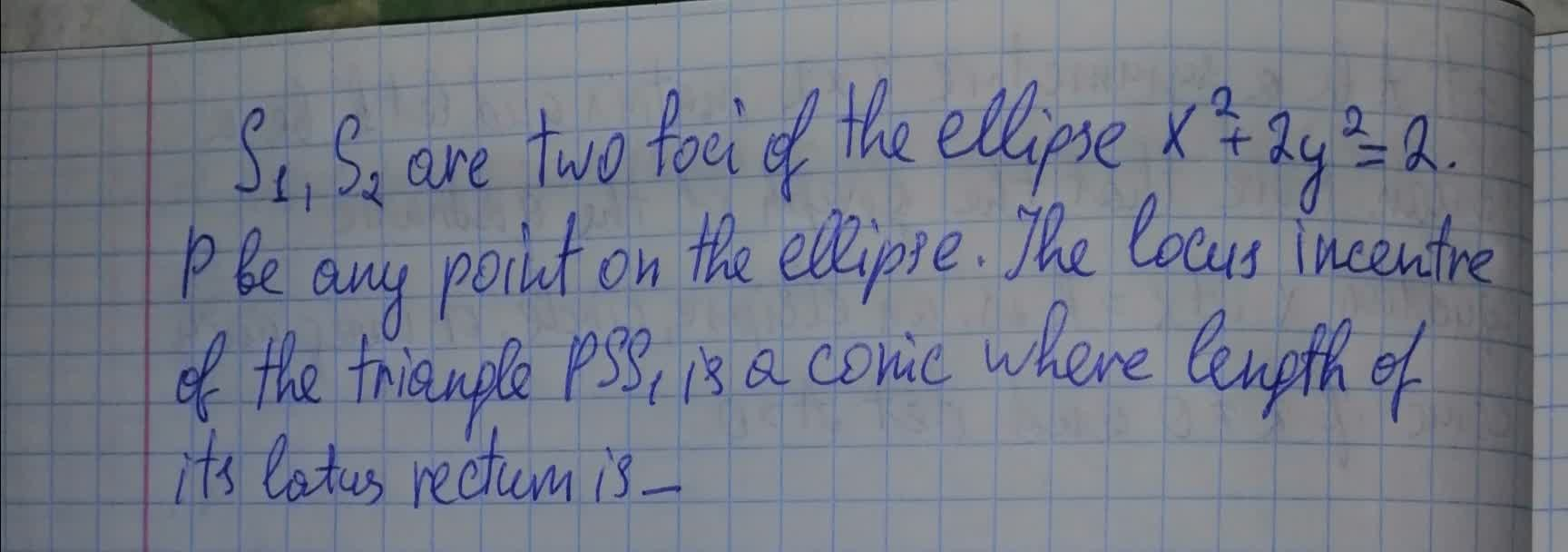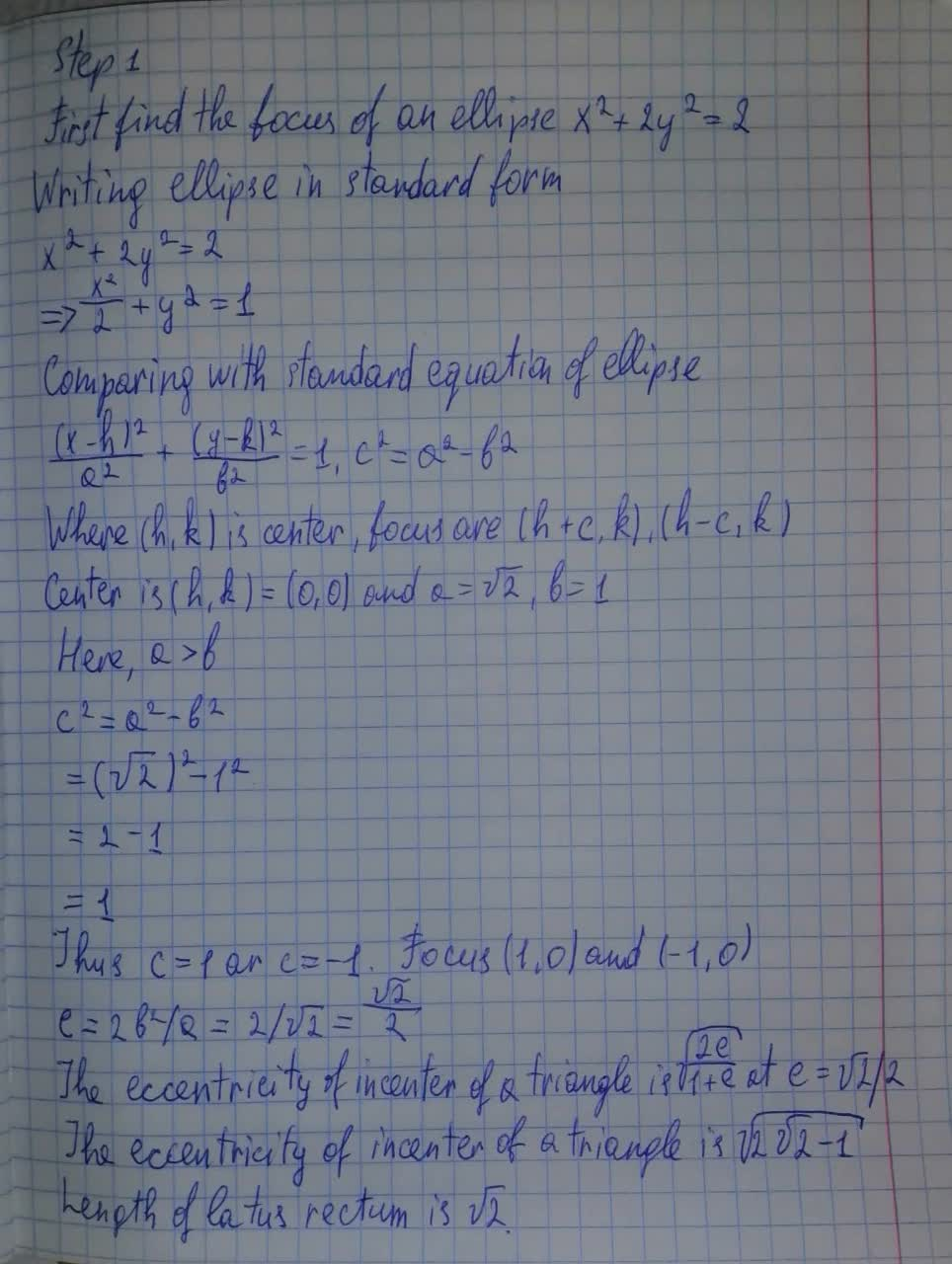# S_1, S_2 are two foci of the ellipse x^2+2y^2 = 2. P be any point on the ellipse The locus incentre of the triangle PSS_1Tazmin Horton 2021-08-11 Answered
$$\displaystyle{S}_{{1}},{S}_{{2}}$$ are two foci of the ellipse $$\displaystyle{x}^{{2}}+{2}{y}^{{2}}={2}$$. P be any point on the ellipse The locus incentre of the triangle $$\displaystyle{P}{S}{S}_{{1}}$$, is a conic where length of its latus rectum is• Questions are typically answered in as fast as 30 minutes

### Plainmath recommends

• Get a detailed answer even on the hardest topics.
• Ask an expert for a step-by-step guidance to learn to do it yourself.liannemdh